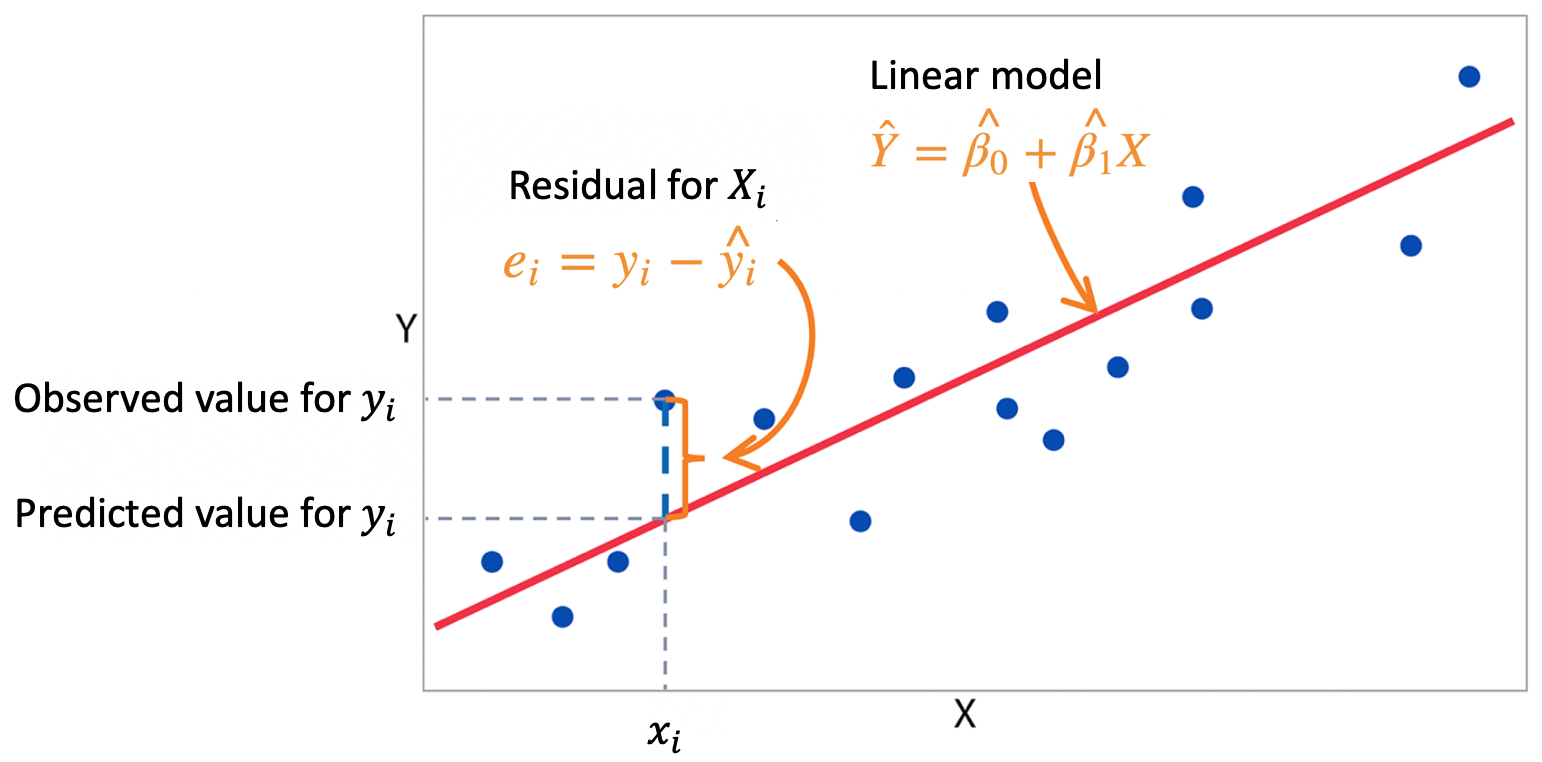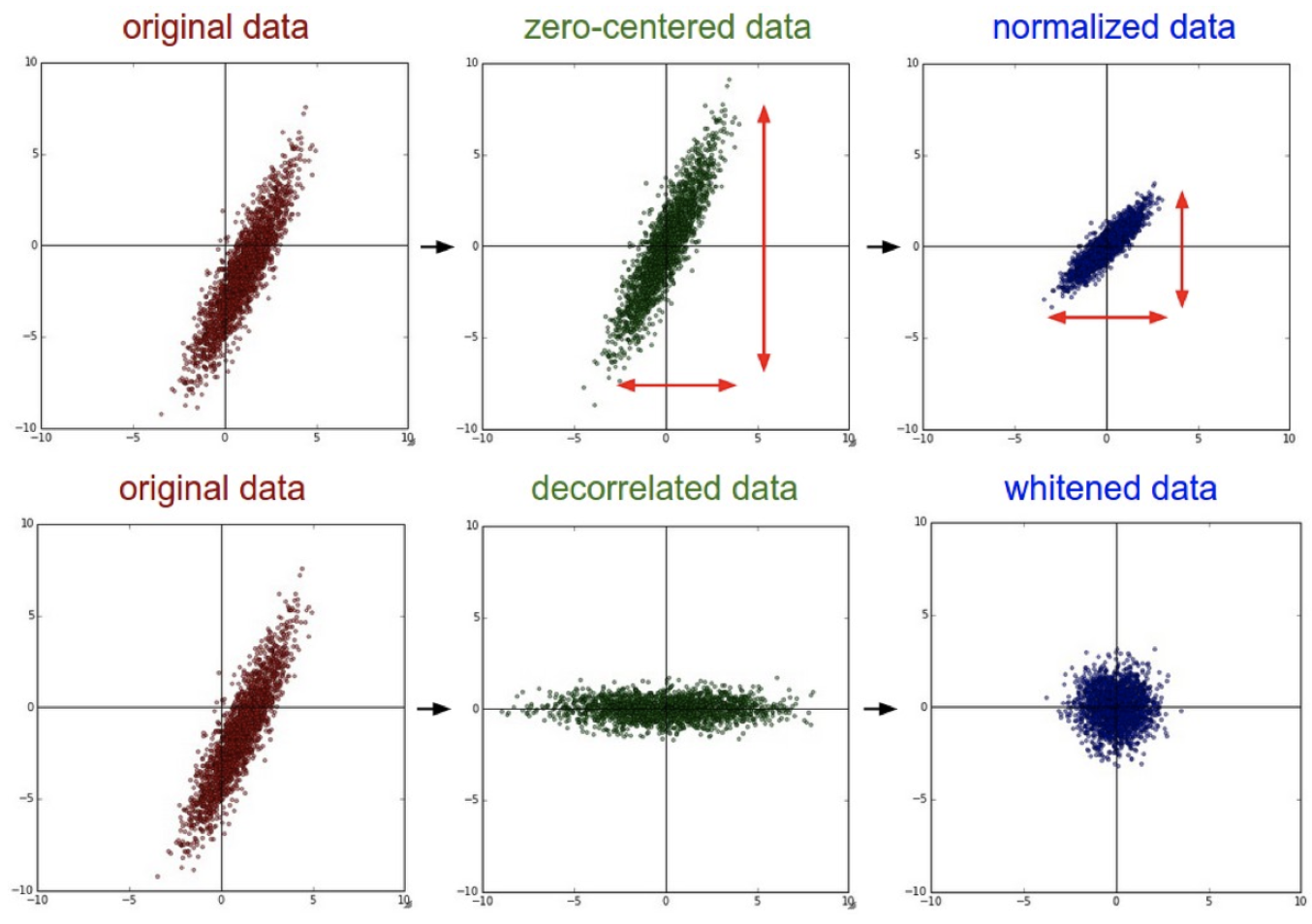# 数据科学统计建模 02：导论 (2)

## 墨尔本大学 MAST90139 课程笔记

Posted by YEY on March 4, 2020

# Lecture 02 导论 (2)

## 4. 拟合一个关于 gavote 的线性模型

### 4.1 最小二乘估计

$\hat{\boldsymbol \beta}=(X^{\mathrm{T}}X)^{-1}X^{\mathrm{T}}\mathbf y$$\mathsf{undercount}=\beta_0+\beta_1\mathsf{pergore}+\beta_2 \mathsf{perAA}+\varepsilon$

R 代码：

1
lmod <- lm(undercount ~ pergore + perAA, gavote); coef(lmod)


1
2
(Intercept)     pergore       perAA
0.03237600  0.01097872  0.02853314


$\hat \beta_0=0.03237600,\quad \hat \beta_1=0.01097872,\quad \hat \beta_0=0.02853314$

### 4.3 相关概念

R 代码：

1
predict(lmod)


1
2
APPLING      ATKINSON         BACON         BAKER       BALDWIN         BANKS  ...
0.04133661    0.04329088    0.03961823    0.05241202    0.04795484    0.03601558  ...


R 代码：

1
residuals(lmod)


1
2
APPLING      ATKINSON         BACON         BAKER       BALDWIN         BANKS  ...
3.694660e-02 -6.994927e-03  6.555058e-02  2.348407e-03  3.589940e-03  1.426726e-02  ...


R 代码：

1
deviance(lmod)


1
 0.09324918


$df(y_1,\dots,y_n;\text{independent})=n$

$df(y_1-\mu_1,\dots,y_n-\mu_n)=n$

$df(y_1-\overline y,\dots,y_n-\overline y)=n-1$

$\hat{\boldsymbol \beta}=(X^{\mathrm{T}}X)^{-1}X^{\mathrm{T}}\mathbf y$

$df(y_1-\hat y_1,\dots,y_n-\hat y_n)=n-p$

R 代码：

1
df.residual(lmod)


1
 156


R 代码：

1
nrow(gavote)-length(coef(lmod))


1
 156


$\hat \sigma=s=\sqrt{\dfrac{\mathsf{RSS}}{\mathsf{df}}}=\sqrt{\dfrac{\sum_{i=1}^{n}(y_i-\hat y_i)^2}{n-p}}$

$\sqrt{\dfrac{\sum_{i=1}^{n}(y_i-\hat y_i)^2}{n-p}}=\sqrt{\dfrac{\sum_{i=1}^{n}(y_i-\overline y)^2}{n-1}}=s$

R 代码：

1
sqrt(deviance(lmod)/df.residual(lmod))


1
 0.02444895


R 代码：

1
lmodsum <- summary(lmod); lmodsum$sigma  输出结果： 1  0.02444895  偏差（deviance） 衡量的是模型在绝对意义上的拟合程度，而不是相对意义上的。一般而言，使用相对差异会更好一些，因为相对差异是无量纲的（dimensionless），我们不需要考虑量纲的影响。模型的相对拟合优度（relative goodness-of-ﬁt）由 决定系数（coeﬃcient of determination），也称解释方差百分比（percentage of variance explained），$R^2$衡量： $R^2=1-\dfrac{\sum_{i=1}^{n}(y_i-\hat y_i)^2}{\sum_{i=1}^{n}(y_i-\overline y_i)^2}=1-\dfrac{\mathsf{RSS}}{\mathsf{TSS}}$ 其中， RSS 是模型残差平方和（residual sum of squares）；而 TSS 是完全平方和（total sum of squares），它等于观测值$\mathbf y$和样本均值之差的平方和。我们可以将 RSS 视为残差的完全方差，将 TSS 其视为观测值$\mathbf y$的完全方差。所以，二者之比$\frac{\mathsf{RSS}}{\mathsf{TSS}}$代表了观测值$\mathbf y$的完全方差中由残差解释的部分所占的比重。而$(1-\frac{\mathsf{RSS}}{\mathsf{TSS}})$则代表了观测值$\mathbf y$的完全方差中可以由模型解释的部分所占的比重，我们将其称为决定系数（coeﬃcient of determination），记为$R^2$，和偏差（deviance）相比，它是一个相对度量。$R^2$也等于$\hat {\mathbf y}$和$\mathbf y$之间相关系数的平方。 关于这点可以通过线性代数相关知识进行证明，不过，更重要的是如何从统计学的直觉上来理解它。我们用$\hat {\mathbf y}$来估计$\mathbf y$，所以二者必须是相关的，因为如果我们用一些和$\mathbf y$毫不相关的东西来预测它从道理上讲不通，所以一般我们会选择和$\mathbf y$最相关的一些东西来预测它。因为拟合值$\hat {\mathbf y}$是从模型中得出的，而决定系数$R^2$衡量了该模型的拟合优度，所以$\hat {\mathbf y}$和$\mathbf y$之间的实际相关系数应当与$R^2$存在某种关联。 R 代码： 1 summary(lmod)$r.squared


1
 0.05308861


R 代码：

1
cor(predict(lmod),gavote$undercount)^2  输出结果： 1  0.05308861  虽然决定系数$R^2$是一个相对度量，但是仍然存在一些局限。可以看到，我们现在拟合的模型有 3 个参数（$\beta_0,\beta_1,\beta_2$）和 2 个预测变量（支持 Gore 的选民占比 pergore 和非裔美国人占比 perAA ）。假如我们在模型中加入更多的预测变量$X$，模型的 RSS 也将变得更小，这样就可以使决定系数$R^2$越来越大。但是由于存在过拟合的风险，引入过多的预测变量$X$并不是一个好的选择，因为这将导致模型预测能力的下降。可见，决定系数$R^2$并不能帮我们修正模型的预测能力，我们需要对$R^2$进行修正。 为此，我们引入 修正决定系数（adjusted coeﬃcient of determination），记为$R_a^2$。它并不用于模型拟合优度的衡量，而是作为模型（预测变量）选择的标准之一。可以看到，它是通过在$R^2$的基础上对分子分母项分别除以各自的自由度得到： $R_a^2=1-\dfrac{\sum_{i=1}^{n}(y_i-\hat y_i)^2/(n-p)}{\sum_{i=1}^{n}(y_i- \overline y_i)^2/(n-1)}=1-\dfrac{\mathsf{RSS}/(n-p)}{\mathsf{TSS}/(n-1)}$ R 代码： 1 summary(lmod)$adj.r.squared


1
 0.04094872


• 对于决定系数 $R^2$：随着参数数量 $p$ 的增加，$R^2$ 将单调递增，导致的结果就是模型容易引入过多的参数而产生过拟合。
• 而对于修正决定系数 $R_a^2$：初始时，$p$ 很小，此时 $R_a^2$ 将随着 $p$ 的继续增加而增大；而当 $p$ 变得很大时，$R_a^2$ 将随着 $p$ 的继续增加而减小。这样，我们就能够为模型选择合适的参数数量 $p$。

### 4.4 查看模型拟合结果

R 代码：

1
2
lmodsum <- summary(lmod);
attributes(lmodsum)


1
2
3
4
5
6
7
$names  "call" "terms" "residuals" "coefficients" "aliased"  "sigma" "df" "r.squared" "adj.r.squared" "fstatistic"  "cov.unscaled"$class
 "summary.lm"


R 代码：

1
summary(lmod)


1
2
3
4
5
6
7
8
9
10
11
12
13
14
15
16
17
18
Call:
lm(formula = undercount ~ pergore + perAA, data = gavote)

Residuals:
Min        1Q    Median        3Q       Max
-0.046013 -0.014995 -0.003539  0.011784  0.142436

Coefficients:
Estimate Std. Error t value Pr(>|t|)
(Intercept)  0.03238    0.01276   2.537   0.0122 *
pergore      0.01098    0.04692   0.234   0.8153
perAA        0.02853    0.03074   0.928   0.3547
---
Signif. codes:  0 ‘***’ 0.001 ‘**’ 0.01 ‘*’ 0.05 ‘.’ 0.1 ‘ ’ 1

Residual standard error: 0.02445 on 156 degrees of freedom
Multiple R-squared:  0.05309,	Adjusted R-squared:  0.04095
F-statistic: 4.373 on 2 and 156 DF,  p-value: 0.01419


R 代码：

1
sumary(lmod)


1
2
3
4
5
6
Estimate Std. Error t value Pr(>|t|)
(Intercept) 0.032376   0.012761  2.5370  0.01216
pergore     0.010979   0.046922  0.2340  0.81531
perAA       0.028533   0.030738  0.9283  0.35470

n = 159, p = 3, Residual SE = 0.02445, R-Squared = 0.05


## 5. 线性模型中的定性 / 分类预测变量

R 代码：

1
2
3
4
5
6
7
contr.helmert(n, contrasts = TRUE, sparse = FALSE)
contr.poly(n, scores = 1:n, contrasts = TRUE, sparse = FALSE)
contr.sum(n, contrasts = TRUE, sparse = FALSE)
contr.treatment(n, base = 1, contrasts = TRUE, sparse = FALSE)
contr.SAS(n, contrasts = TRUE, sparse = FALSE)

gavote$cperAA <- gavote$perAA - mean(gavote$perAA) lmodi <- lm(undercount ~ cperAA+cpergore*usage+equip, gavote) sumary(lmodi)  输出结果： 1 2 3 4 5 6 7 8 9 10 11 12 Estimate Std. Error t value Pr(>|t|) (Intercept) 0.0432973 0.0028386 15.2529 < 2.2e-16 cperAA 0.0282641 0.0310921 0.9090 0.364786 cpergore 0.0082368 0.0511562 0.1610 0.872299 usageurban -0.0186366 0.0046482 -4.0095 9.564e-05 equipOS-CC 0.0064825 0.0046799 1.3852 0.168060 equipOS-PC 0.0156396 0.0058274 2.6838 0.008097 equipPAPER -0.0090920 0.0169263 -0.5372 0.591957 equipPUNCH 0.0141496 0.0067827 2.0861 0.038658 cpergore:usageurban -0.0087995 0.0387162 -0.2273 0.820515 n = 159, p = 9, Residual SE = 0.02335, R-Squared = 0.17  我们来看一下模型 lmodi 的参数数量$p$应该是多少： • 首先，截距项 Intercept 包含 1 个参数； • 然后，数值变量 cperAAcpergore 各包含 1 个参数； • 接下来，因子 usage 包含 2 个 levels（ruralurban），所以这里将 rural 作为 base level，再用 1 个虚拟变量 usageurban 来编码 usage，因此也包含 1 个参数； • 同理，因子 equip 包含 5 个 levels，将第 1 个作为 base level，然后用 4 个虚拟变量（equipOS-CC, equipOS-PC, equipPAPERequipPUNCH ），所以包含 4 个参数； • 最后，对于截距项 cpergore:usage，由于 usage 已经用虚拟变量 usageurban 编码过了，所以它也被编码为 cpergore:usageurban，所以也包含 1 个参数。 所以，该模型的参数数量为：$p=1+1+1+1+4+1=9模型 lmodi 的数学形式为： \begin{align} \mathsf{undercount} = &\, \beta_0+\beta_1 \mathsf{cperAA}+\beta_2 \mathsf{cpergore}+\beta_3 \mathsf{usageurban}\\ &\, +\beta_4 \mathsf{equipOSCC}+\beta_5 \mathsf{equipOSPC}+\beta_6 \mathsf{equipPAPER}\\ &\, +\beta_7 \mathsf{equipPUNCH}+\beta_8 \mathsf{cpergore}\times \mathsf{usageurban} +\varepsilon \end{align} 其中，如果 usage=$urban，那么虚拟变量 usaageurban$=1$；如果 usage$\ne$urban，那么 usaageurban$=0。其他的虚拟变量如 equipOS-CC 等皆同理。 模型 lmodi 的估计（estimate）为： \begin{align} \widehat{\mathsf{undercount}} = &\, 0.043+0.028\, \mathsf{cperAA}+0.008\, \mathsf{cpergore}-0.019\, \mathsf{usageurban}\\ &\, +0.006\, \mathsf{equipOSCC}+0.016\, \mathsf{equipOSPC}-0.009\, \mathsf{equipPAPER}\\ &\, +0.014\, \mathsf{equipPUNCH}-0.009\, \mathsf{cpergore}\times \mathsf{usageurban} \end{align} 参数\boldsymbol \beta$的估计（estimates）的标准误（standard errors）可以通过 sumary(lmodi) 查看。 如果建模时还引入了交互项 cpergore:equip，那么模型还会增加 4 个额外的交互项：cpergore:equipOS-CC$,…,$cpergore:equipPUNCH ### 6.2 模型解释 考虑一个 usage 的 level 为 rural 的郡，该郡支持 Gore 的选民占比 pergore 和非裔美国人占比 perAA 在所有的郡中处于平均水平，另外，该郡采用的投票机器 equip 型号为 LEVER R 代码： 1 sumary(lmodi)  输出结果： 1 2 3 4 5 6 7 8 9 10 11 12 Estimate Std. Error t value Pr(>|t|) (Intercept) 0.0432973 0.0028386 15.2529 < 2.2e-16 cperAA 0.0282641 0.0310921 0.9090 0.364786 cpergore 0.0082368 0.0511562 0.1610 0.872299 usageurban -0.0186366 0.0046482 -4.0095 9.564e-05 equipOS-CC 0.0064825 0.0046799 1.3852 0.168060 equipOS-PC 0.0156396 0.0058274 2.6838 0.008097 equipPAPER -0.0090920 0.0169263 -0.5372 0.591957 equipPUNCH 0.0141496 0.0067827 2.0861 0.038658 cpergore:usageurban -0.0087995 0.0387162 -0.2273 0.820515 n = 159, p = 9, Residual SE = 0.02335, R-Squared = 0.17  由于 ruralLEVER 是作为 usageequip 这两个分类变量的 reference levels（即 base levels），因此，它们对于 undercount 的预测值并没有什么贡献。另外，由于我们已经对数值变量 pergoreperAA 在各自的均值上进行了中心化处理，所以这两个变量也不会被引入模型之中。注意，中心化的意义在于：如果没有经过中心化处理，那么我们需要将这些变量设置为$0$，使其脱离预测方程; 而$0$并不是这些预测变量的典型值。假设所有其他项均被删除，则 undercount 的预测值仅由截距项（intercept）$\hat \beta_0$给出，即$4.33\%$。图 1：几种数据预处理的可视化说明：零-中心化、归一化、去相关化、白化 现在可以相对于该基准来对系数进行解释。我们看到，在所有其他预测变量都不变的情况下，除了使用具有区域计数的光学扫描设备（OS-PC）之外，预测的 undercount 增加了$1.56\%$。其他的 equip 型号也可以采用类似的解释。请注意，我们需要对这种解释保持谨慎。给定两个郡，它们除了投票设备 equip 不同之外，其他的预测变量的取值都一样，我们 预测 采用OS-PC 的郡的 undercount 将比采用 LEVER 的郡的 undercount 的值高出$1.56\%$。但是，我们不能这么说：如果我们去了一个采用 LEVER 的郡，并将其更改为 OS-PC，就将使 undercount 增加$1.56\%$。 在所有其他预测变量保持不变的情况下，我们可以预测某个郡从没有非洲裔美国人的情况转变到全部为非洲裔美国人的情况时，undercount 将增长$2.83\%$。有时一个单位的预测变量的变化可能太大或太小，我们可以对解释进行重新调整。例如，对于非裔美国人比例增长了$10\%$的情况，我们可以预测 undercount 将增加$0.283\%$。当然，这种解释不应该太当真。请注意，我们已经知道非裔美国人占比 perAA 和支持 Gore 的选民占比 pergore 之间强相关，因此，其中某一方占比上升将导致另一方占比也随之上升。这种被称为 共线性（collinearity） 的问题将使得对回归系数的解释更加困难。并且，非裔美国人的比例 perAA 很可能与其他社会经济变量有关，而这些变量也可能与 undercount 有关。这进一步阻碍了得出一个 因果结论（causal conclusion） 的可能性。 对于 usagepergore 这两个变量的解释不能被割裂开来，因为在模型 lmodi 中存在一个这两者的交互项。我们可以看到，对于一个支持 Gore 选民占比的平均数字，我们预测一个 urban 的郡的 undercount 会比一个 rural 的郡要低$1.86\%$。在一个 rural 的郡，我们预测随着 Gore 支持者占比 pergore 上升$10\%$，undercount 将上升$0.08\%$。而在一个 urban 的郡，我们预测随着 pergore 上升$10\%$，undercount 将上升$(0.00824-0.00880)\times 10=-0.0056\%\$，而一个负增长实际上就意味着下降。这说明当某个变量存在一个与之相关的交互项时，我们在对该变量的效应进行预测时必须小心一些 潜在的陷阱。我们不能对 Gore 支持者占比 pergore 对于响应变量 undercount 的效应直接给出一个简单独立的解释。事实上，pergore 这个变量的效应体现在：对于 rural 的郡，undercount 会随着该变量的上升而上升；而对于 urban 的郡，undercount 则会随着该变量的上升而下降。本作品采用知识共享署名-非商业性使用-相同方式共享 4.0 国际许可协议进行许可。 欢迎转载，并请注明来自：YEY 的博客 同时保持文章内容的完整和以上声明信息！